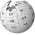# MATLAB您可以在Wikipedia上了解到此条目的英文信息 MATLAB Thanks, Wikipedia.

MATLAB是MATrix LABoratory的缩写，是一款由美国MathWorks公司出品的商业数学软件。MATLAB 是一种用于算法开发、数据可视化、数据分析以及数值计算的高级技术计算语言和交互式环境。

GNU OctaveScilab 是 MATLAB 的开源替代产品，大家可试试。